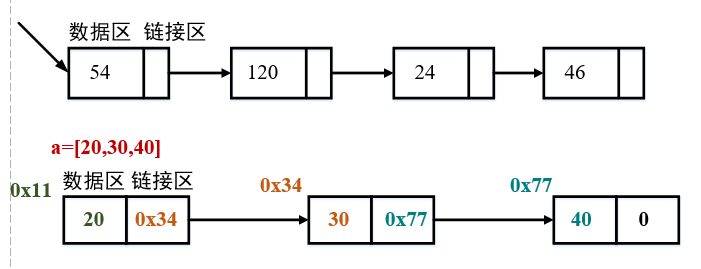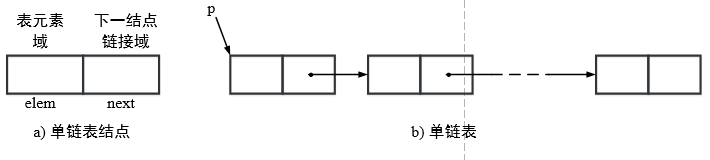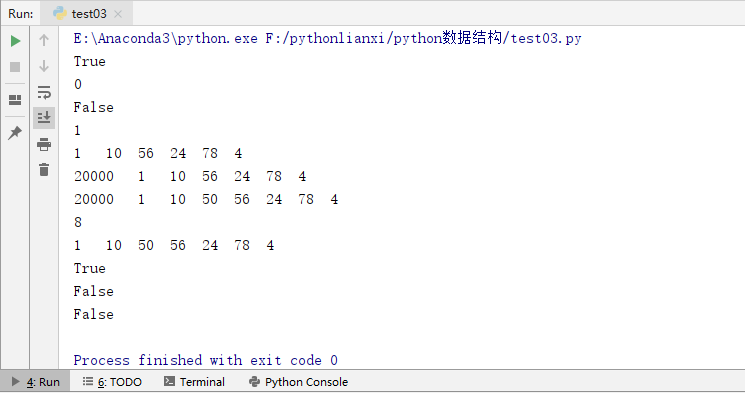# 线性表(单向链表(动图解析))1148-张同学

,

## 链表的定义## 单向链表1. 表元素域elem用来存放具体的数据。
2. 链接域next用来存放下一个节点的位置（python中的标识）
3. 变量p指向链表的头节点（首节点）的位置，从p出发能找到表中的任意节点。
通过例子理解

## 节点实现

// An highlighted block
"""单链表的结点"""
def __init__(self,item=None,node=None):
self.item = item
self.next = None


## 单链表的操作

##### 判断链表是否为空(is_empty())// An highlighted block
"""判断链表是否为空"""
def is_empty(self):

##### 计算链表长度(length())// An highlighted block
"""链表长度"""
def length(self):
count = 0
while cur != None: # 尾节点指向None，当未到达尾部时
count += 1
cur = cur.next # 将cur后移一个节点
return count

##### 遍历整个链表(travel() )

// An highlighted block
"""遍历链表"""
def travel(self):
while cur != None:
print(cur.item,end="/t")
cur = cur.next
print(end="/n")

##### 尾部添加元素(尾插法)// An highlighted block
"""尾部添加元素"""
def append(self, item):
node = SingleNode(item)
else:
while cur.next != None:  #判断下一个节点是否为None
cur = cur.next
cur.next = node

##### 头部添加元素(头插法)// An highlighted block
"""头部添加元素"""
node = SingleNode(item)

##### 在指定位置添加节点// An highlighted block
"""在指定位置添加元素

"""
def insert(self, pos, item):
#判断指定位置是进行头部添加还是尾部添加
if pos <= 0:
elif pos > (self.length() - 1):
self.append(item)
else:
node = SingleNode(item)
count = 0
# 通过计数判断是否移动到指定位置的前一个位置
while count<(pos - 1):
count+=1
cur = cur.next
node.next = cur.next
cur.next = node

##### 查找节点是否存在// An highlighted block
"""查找节点是否存在"""
def search(self, item):
while cur != None:
if cur.item == item:
print("True")
return True
else:
cur = cur.next
print("False")
return False

##### 删除一个节点// An highlighted block
"""删除一个节点"""
def remove(self, item):
# 若链表为空，则直接返回
pre = None
# 若头节点的元素就是要查找的元素item
while cur!= None:
if cur.item==item:
#先判断此节点是否为头结点
else:
pre.next=cur.next
break
else:
pre=cur
cur=cur.next


## 程序运行

// An highlighted block
if __name__=="__main__":
print(a.is_empty())
print(a.length())

#在尾部增加元素
a.append(1)
print(a.is_empty())
print(a.length())
a.append(10)
a.append(56)
a.append(24)
a.append(78)
a.append(4)
a.travel()
#在头部增加元素
a.travel()
# 在指定位置插入
a.insert(3,50)
a.travel()
print(a.length())
#删除元素
a.remove(20000)
a.travel()
#查找元素
a.search(50)
a.search(10000)
a.search(30000)### 评论 抢沙发### 觉得文章有用就打赏一下文章作者

#### 支付宝扫一扫打赏#### 微信扫一扫打赏Vieu3.3主题Q Q 登 录